cancel
Showing results for
Did you mean: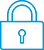It has been a while since anyone has replied. Simply ask a new question if you would like to start the discussion again.New member
3 2 0 0
Message 1 of 5
740
Flag Post

Solved!

# The HP Prime can't factor complex equations and struggles with other stuffHP Recommended
Hp Prime

The Prime seems to have a really hard time factoring complex polynomials such as X^3 - X^2 +X +1 for instance. If anyone knows how to factor this on a Prime, let me know. I really wanted to like the Prime more than my 89 and/or Nspire, because it's just stupid fast spitting out answers when doing complicated operations such as summation  Unfortunately, the 2 TIs can do so much more stuff that the HP can't, surprisingly.

Tags (4)
1 ACCEPTED SOLUTION

Accepted SolutionsLevel 5
114 113 26 45
Message 4 of 5
Flag PostHP Recommended

Hi Gatra,

May I suggest the following method for solving for x for Sin(x)=sqrt(2)/2=Cos(x)?

First you need to go in to CAS Settings and untick Principle: in first page as in the picture.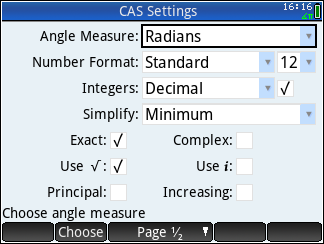then you issue the command solve((sin(x)) = ((cos(x)) = (sqrt(2)/2)))

The Prime will give you following answer: {(1/4)*(8*n_5*π+π),(1/4)*(8*n_5*π-π)}. Here the n_5 could vary such as  n_1, n_2.. etc. Depending on your previous CAS session. This n_5, in my case, refers to all set of integers -n to n.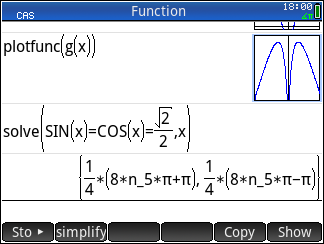So, to check the actual values of n_5 in a sample range of say -2 to 2, against a given answers, you do the following;

issue the command (for the fist value of answers in set) seq((1/4)*(8*n_5*π+π),n_5 = ((-2) .. 2),1) which will give following set answers:  The principle/primary value of x is obviously  π/4. as it's first value close to 0.

[(-15/4)*π,(-7/4)*π,(1/4)*π,(1/4)*9*π,(1/4)*17*π]As regards to your second question, you've answered it yourself 😁. The prime doesn't know what you wanted to do with TAN(x). until you've specified trigsin(TAN(x)).

Not trying to be patronizing, but it will help you in long run to read up on the settings of HOME, CAS, PLOT SYMB and NUM settings.

Tags (1)
4 REPLIES 4Level 8
650 635 124 222
Message 2 of 5
Flag PostHP Recommended

That particular polynomial has no factors.  If you are looking for its zeros, use the zeros( ) function.  Depending on your CAS settings, the output will be only the real zeros or both the real and complex zeros.

If your other non-HP calculators return factors for that polynomial, please let us know what they are.  If they return 'X+0.543689012692' or similar, that's not really correct.  It's only an approximation, based on the approximated root of f(x)=0.  If that's what you want, HP Prime offers that via the zeros( ) function, as well as other solver functions such as froot( ).

Disclaimer: I don't work for HP. I'm just a happy HP calculator user.

-Joe-New member
3 2 0 0
Message 3 of 5
Flag PostHP Recommended

You're absolutely right! I was comparing calculators late at night and I wasn't thorough enough. Though, I did type in x3-x2-x+1 on the TIs, and for whatever reason failed to input the same exact expression on the prime! If I'd paid attention and put -x rather than x, this post wouldn't have existed lol.

However, I hit another wall while trying to solve this simple system of equation. Any idea?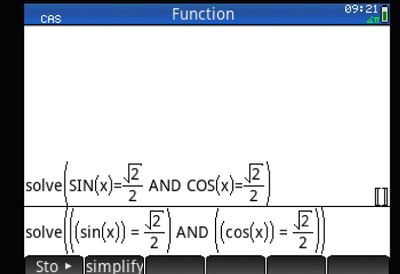Moreover, the only way I could simplify TAN to be sin/cos was to go to catalog and select functions like trigsin, trigcos, etc.... The expand functions do not seem to work with just tan.

Interestingly enough, the calculator has no problem simplifying reciprocal functions as pictured. I find this quite unfortunate.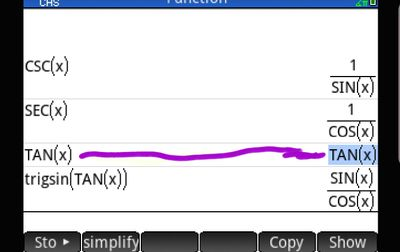Tags (5)Level 5
114 113 26 45
Message 4 of 5
Flag PostHP Recommended

Hi Gatra,

May I suggest the following method for solving for x for Sin(x)=sqrt(2)/2=Cos(x)?

First you need to go in to CAS Settings and untick Principle: in first page as in the picture.then you issue the command solve((sin(x)) = ((cos(x)) = (sqrt(2)/2)))

The Prime will give you following answer: {(1/4)*(8*n_5*π+π),(1/4)*(8*n_5*π-π)}. Here the n_5 could vary such as  n_1, n_2.. etc. Depending on your previous CAS session. This n_5, in my case, refers to all set of integers -n to n.So, to check the actual values of n_5 in a sample range of say -2 to 2, against a given answers, you do the following;

issue the command (for the fist value of answers in set) seq((1/4)*(8*n_5*π+π),n_5 = ((-2) .. 2),1) which will give following set answers:  The principle/primary value of x is obviously  π/4. as it's first value close to 0.

[(-15/4)*π,(-7/4)*π,(1/4)*π,(1/4)*9*π,(1/4)*17*π]As regards to your second question, you've answered it yourself 😁. The prime doesn't know what you wanted to do with TAN(x). until you've specified trigsin(TAN(x)).

Not trying to be patronizing, but it will help you in long run to read up on the settings of HOME, CAS, PLOT SYMB and NUM settings.

Tags (1)New member
3 2 0 0
Message 5 of 5
Flag PostHP Recommended

This was very helpful; thanks a bunch! Mastering this calculator is definitely going to take me some time,  and I'll get there 🙂 👍

† The opinions expressed above are the personal opinions of the authors, not of HP. By using this site, you accept the Terms of Use and Rules of Participation